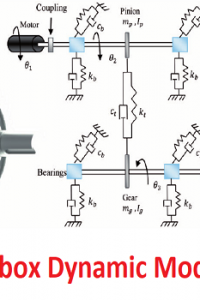اسم المؤلف
research
التاريخ
22 يوليو 2021
المشاهدات
253
التقييم(لا توجد تقييمات)Loading...

بحث بعنوان
Gearbox Dynamic Modelling
Walter Bartelmus
Machinery Systems Division, Wrocław University of Technology
The paper reports on current developments in gearbox dynamic modelling. It refers to M¨uller’s one-mass two-parameter (stiffness and damping) gearbox model with rectilinear vibration. The paper shows that
there is a need to develop a new model, which would incorporate torsional vibration. The paper refers to the previous papers on gearbox
proceedings. In the present paper, the influence of the clutch damping
coefficient and one random parameter value from the three-parameter
error mode, and that of the interaction between error parameters on the
vibration generated by a gearbox system, is analyzed.
Key words: gearbox model, mathematical modelling, computer simulation.
Introduction
The modelling of gearbox dynamic behaviour belongs to the fundamental
problems of mechanical system modelling. The problem has been the subject
of many papers in Poland and abroad: Wang (1974), Mark (1978), Velex and
Maatar (1996), Smith (1998). The model presented by M¨uller in 1979 has
received a lot of attention in Poland. It was discussed, for example, by Ryś
(1977), Wilk (1981), and Dąbrowski (1992). The model is a two-parameter
(stiffness and damping) model in which the inertia of two wheels has been
reduced to one mass equivalent to a one-stage gearbox.
The author of the present paper has found that more sophisticated models are needed to describe the gearbox dynamics properly; Bartelmus (1994,
2000). Mathematical modelling and computer simulation can be applied to990 W. Bartelmus
gearbox dynamics to support diagnostic signal evaluation for diagnostic inference. This is the main aim of the present research. The computer simulation is
based on a mathematical model developed by Bartelmus (1994, 2000). General
information on gearing needed for the computer simulation of gearbox behaviour was given by Bartelmus (2000). Some results of computer simulations
supporting diagnostic inference were presented by Bartelmus (1996-1999). The
papers show that mathematical modelling and computer simulation enable the
detailed investigation of the dynamic properties of a gearing system. All the
basic factors such as: design, technology, operation and change of the gearing
system conditions which have a bearing on the vibration generated by a gearing can be investigated. The causes of vibration in gearboxes are mainly the
tooth errors and vibration is an indication of them. The computer simulation
results are referred to the laboratory investigation results presented by Rettig
(1977) and to the field measurements reported by Penter (1991), Bartelmus
(1992), and Tuma et al. (1994).
As mentioned above, the vibration of a gearbox indicates that there are
tooth errors in it. The errors appear at the production stage. The nature of
the gear-wheel interaction is such that non-linear phenomena occur caused by
friction, intertooth backlash, impact-like intertooth forces and periodic changes in tooth stiffness. As a result, the intertooth forces may exceed the force
values, which follow from the gearbox system’s rated moment. Mathematical
description enabling to include these phenomena in the equation of motion is
given by Bartelmus (2000).
The intertooth forces increase dramatically under unstable conditions. A
one-stage gear system operates under resonance conditions and is unstable
when the gearbox system’s mesh frequency is equal to its natural frequency.
In such conditions, the intertooth forces are two times greater than the rated forces. The phenomenon of resonance has not been investigated fully for
gearbox systems.
Computer simulations reveal that conditions similar to the ones occurring
at resonance may result as errors (pitting, scuffing of teeth flanks and failure of
bearings) increase during the service of a gearbox system. In the present paper,
current developments in gearbox modelling are presented with reference to the
previous papers by the present author. It is shown that a flexible clutch and an
error mode random parameter have an influence on the gearbox stability (teeth
separation). An error mode is described by several parameters, i.e. maximum
error value, shape of error plot and random error fluctuation depth.
Conclusions
The paper has shown a detailed analysis of a mutual influence of the error
mode parameters and influence of a clutch damping on a dynamic factor Kd,
which reveals the gearing condition. The error mode is described by three
parameters: a – shape parameter, e – maximum value of the error, r – scope998 W. Bartelmus
of the error random change. The analysis proves that, in general conditions
of a gearing change (change of e and r), the linear increase of Kd does not
hold, as it is stated in Bartelmus (1992) (experiment on a real object) and
1996 (computer simulation experiment). In the experiment on a real object
and in the computer simulation experiment, only the value of e was taken
into consideration.
كلمة سر فك الضغط : books-world.net